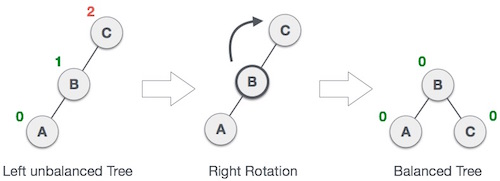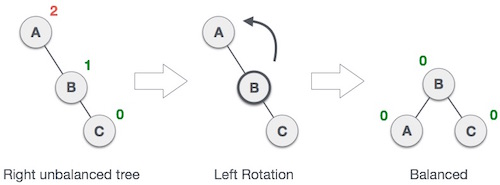# Algorithms -- AVL Trees

An AVL tree is a subtype of binary search tree. Named after it’s inventors Adelson, Velskii and Landis, AVL trees have the property of dynamic self-balancing in addition to all the properties exhibited by binary search trees.

A BST is a data structure composed of nodes. It has the following guarantees:

1. Each tree has a root node (at the top).
2. The root node has zero, one or two child nodes.
3. Each child node has zero, one or two child nodes, and so on.
4. Each node has up to two children.
5. For each node, its left descendents are less than the current node, which is less than the right descendents.

AVL trees have an additional guarantee:

1. The difference between the depth of right and left subtrees cannot be more than one. In order to maintain this guarantee, an implementation of an AVL will include an algorithm to rebalance the tree when adding an additional element would upset this guarantee.

AVL trees have a worst case lookup, insert and delete time of O(log n).

### Right Rotation### Left Rotation### AVL Insertion Process

You will do an insertion similar to a normal Binary Search Tree insertion. After inserting, you fix the AVL property using left or right rotations.

• If there is an imbalance in left child of right subtree, then you perform a left-right rotation.
• If there is an imbalance in left child of left subtree, then you perform a right rotation.
• If there is an imbalance in right child of right subtree, then you perform a left rotation.
• If there is an imbalance in right child of left subtree, then you perform a right-left rotation.

An AVL tree is a self-balancing binary search tree. An AVL tree is a binary search tree which has the following properties: ->The sub-trees of every node differ in height by at most one. ->Every sub-tree is an AVL tree.

AVL tree checks the height of the left and the right sub-trees and assures that the difference is not more than 1. This difference is called the Balance Factor. The height of an AVL tree is always O(Logn) where n is the number of nodes in the tree.

AVL Tree Rotations:-

In AVL tree, after performing every operation like insertion and deletion we need to check the balance factor of every node in the tree. If every node satisfies the balance factor condition then we conclude the operation otherwise we must make it balanced. We use rotation operations to make the tree balanced whenever the tree is becoming imbalanced due to any operation.

Rotation operations are used to make a tree balanced.There are four rotations and they are classified into two types: ->Single Left Rotation (LL Rotation) In LL Rotation every node moves one position to left from the current position. ->Single Right Rotation (RR Rotation) In RR Rotation every node moves one position to right from the current position. ->Left Right Rotation (LR Rotation) The LR Rotation is combination of single left rotation followed by single right rotation. In LR Rotation, first every node moves one position to left then one position to right from the current position. ->Right Left Rotation (RL Rotation) The RL Rotation is combination of single right rotation followed by single left rotation. In RL Rotation, first every node moves one position to right then one position to left from the current position.

Time Analysis Of AVL Tree:

AVL tree is binary search tree with additional property that difference between height of left sub-tree and right sub-tree of any node can’t be more than 1.

Algorithm Average Worst case Space O ( n ) {\displaystyle O(n)} O(n) O ( n ) {\displaystyle O(n)} O(n) Search O ( log ⁡ n ) {\displaystyle O(\log n)} O(\log n) O ( log ⁡ n ) {\displaystyle O(\log n)} O(\log n) Insert O ( log ⁡ n ) {\displaystyle O(\log n)} O(\log n) O ( log ⁡ n ) {\displaystyle O(\log n)} O(\log n) Delete O ( log ⁡ n ) {\displaystyle O(\log n)} O(\log n) O ( log ⁡ n ) {\displaystyle O(\log n)} O(\log n)

Application of AVL Trees

AVL trees are beneficial in the cases where you are designing some database where insertions and deletions are not that frequent but you have to frequently look-up for the items present in there.